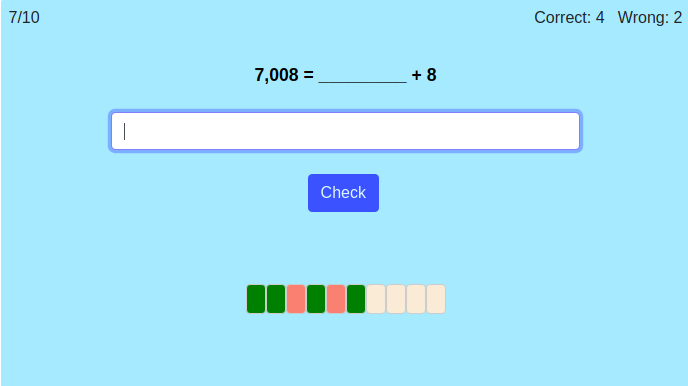MM Practice
×
Multiplication
Division
Place Value
Fractions & Decimals
Measurement
Statistics & Probability
Pre-Algebra
Money
Kindergarten
Geometry

# Place ValueOnline practice for grades 1-4

This script makes simple math problems that help students practice place value concepts.

In the first mode, students need to find the missing part of a number, such as 2,594 = 500 + ______ + 4 + 2,000.

In the second mode, students are given a number in expanded form and they need to write the number. For example, 500 + 30 + 7,000 + 2 makes the number 7,532.

You can choose timed or untimed practice, the number of practice problems, the 'mode', and the maximum number of digits in the number.

Screenshot:OR
Practice for a set time: min

Allow my comment to be posted on this site